### ASM to Sega Genesis Platform

Would you like to react to this message? Create an account in a few clicks or log in to continue.
ASM to Sega Genesis Platform

All about assembly programming in the Sega Genesis console.

5 posters

# Homing Attack - Variant 1 - Distance MethodOuricoDoido

Mensagens : 46
Data de inscrição : 2011-01-09
Current Project : Sonic Open Source Project
Here a Method that not need the CalcAngle and CalcSine, the main difference is the "speed by distance" (near = slow, far = fast). Because how much closer of the object (short distances is a low value), lower is the speed (lower speeds is a low value).To apply this, you need first apply the Homing Attack in Sonic 1 guide.
After apply the guide, follows below.

First in HA_Move delete this:
Code:
`      jsr      (CalcAngle).l   ; calculates the angle      jsr      (CalcSine).l   ; calculates the sine and the cosine`

Again in HA_Move change this:
Code:
`      muls.w  #\$C,d1         ; multiply cosine by \$C      move.w  d1,\$10(a0)      ; move d1 to X-velocity      muls.w  #\$C,d0         ; multiply sine by \$C      move.w  d0,\$12(a0)      ; move d0 to Y-velocity`
To this:
Code:
`      muls.w  #\$10,d1         ; multiply x-distance by \$10      move.w  d1,\$10(a0)      ; move d1 to X-velocity      muls.w  #\$10,d2         ; multiply y-distance by \$10      move.w  d2,\$12(a0)      ; move d2 to Y-velocity`
To the Sonic go more faster, change the \$10 by a higher value.

Now because the "speed by distance", we need more time to hit the enemy; in HA_calcdistance2 change this:
Code:
`      move.b   #30,(\$FFFFFFD1).w   ; number of frames Sonic can chasing the object`
To this:
Code:
`      move.b   #120,(\$FFFFFFD1).w   ; number of frames Sonic can chasing the object`

After applying these changes, your code must be equal to this below:
Code:
`; ---------------------------------------------------------------------------; Subroutine Sonic_Homingattack; ---------------------------------------------------------------------------; ||||||||||||||| S U B   R O U T   I N E |||||||||||||||||||||||||||||||||||||||Sonic_Homingattack:      tst.b   (\$FFFFFFD1).w      ; is Sonic chasing some object?      bne.w   HA_Move            ; if yes, chase him      move.b   (\$FFFFF603).w,d1   ; read controller      andi.b   #\$40,d1            ; is A pressed?      beq.w   Sonic_HA_rts      ; if not, branch      lea      (\$FFFFD800).w,a1   ; start at the first level object RAM; ---------------------------------------------------------------------------HA_enemylist:      tst.b   (a1)         ; is a Null object      beq.s   HA_nextobject   ; if yes, branch      cmpi.b   #5,\$20(a1)      ; is not an enemy object? (spring, explosion, platform, collected ring, flame thrower (SBZ), among others)      bcs.w   HA_nextobject   ; if yes, branch      ; cmpi.b   #5,\$20(a1)      ; is Burrobot enemy (LZ)      ; beq.w   HA_calcdistance   ; if yes, branch      ; cmpi.b   #6,\$20(a1)      ; is Crabmeat enemy (GHZ, SYZ)      ; beq.s   HA_calcdistance   ; if yes, branch      ; cmpi.b   #8,\$20(a1)      ; is Buzz Bomber enemy (GHZ, MZ, SYZ)      ; beq.s   HA_calcdistance   ; if yes, branch      ; cmpi.b   #9,\$20(a1)      ; is Chopper enemy (GHZ)      ; beq.s   HA_calcdistance   ; if yes, branch      ; cmpi.b   #\$A,\$20(a1)   ; is Jaws enemy (LZ)      ; beq.s   HA_calcdistance   ; if yes, branch      ; cmpi.b   #\$B,\$20(a1)   ; is Caterkiller enemy (MZ, SBZ) / Orbinaut enemy (LZ, SLZ, SBZ) / Basaran enemy (MZ)      ; beq.s   HA_calcdistance   ; if yes, branch      ; cmpi.b   #\$C,\$20(a1)   ; is Roller enemy (SYZ) / Newtron enemy (GHZ)      ; beq.s   HA_calcdistance   ; if yes, branch         ; cmpi.b   #\$D,\$20(a1)   ; is Newtron enemy (GHZ)      ; beq.s   HA_calcdistance   ; if yes, branch      ; cmpi.b   #\$E,\$20(a1)   ; is Roller enemy (SYZ)      ; beq.s   HA_calcdistance   ; if yes, branch      ; cmpi.b   #\$F,\$20(a1)   ; is Eggman      ; beq.s   HA_calcdistance   ; if yes, branch      cmpi.b   #\$F,\$20(a1)      ; is some enemy of the list above?      bls.s   HA_calcdistance ; if yes, branch      cmpi.b   #\$46,\$20(a1)   ; is the monitor?      beq.s   HA_calcdistance ; if yes, branch      ; cmpi.b   #\$47,\$20(a1)      ; is the ring?      ; beq.s   HA_calcdistance       ; if yes, branch      cmpi.b   #\$52,\$20(a1)   ; is giant ring for entry to special stage?      beq.s   HA_calcdistance ; if yes, branch      cmpi.b   #\$CC,\$20(a1)   ; is Yadrin enemy (SYZ)      beq.s   HA_calcdistance ; if yes, branchHA_nextobject:      adda.w  #\$40,a1         ; jump to next object RAM entry      cmpa.w  #\$F000,a1      ; already tested all object RAM entry?      blt.s  HA_enemylist   ; if not, return to enemy list      rts; ---------------------------------------------------------------------------HA_calcdistance:   ; distance calculator      move.w  8(a1),d1   ; move the object x-position to d1      move.w  \$C(a1),d2   ; move the object y-position to d2      sub.w  8(a0),d1   ; sub sonic x-position of object x-position      sub.w  \$C(a0),d2   ; sub sonic y-position of object y-position; ---------------------------------------------------------------------------; test if the Sonic is facing the object      btst    #0,\$22(a0)   ; is sonic facing left?      beq.s  HA_faceleft   ; if yes, branch      cmpi.w  #8,d1      ; is distance of Sonic, less than 8 pixels of the object?      blt.s  HA_calcdistance2   ; if yes, branch      bra.s  HA_nextobjectHA_faceleft:      cmpi.w  #-8,d1      ; is distance of Sonic, greater than -8 pixels of the object?      bgt.s  HA_calcdistance2   ; if yes, branch      bra.s  HA_nextobject; end of test; ---------------------------------------------------------------------------HA_calcdistance2:      ; continuation of distance calculator      muls.w  d1,d1   ; horizontal distance squared      muls.w  d2,d2   ; vertical distance squared      add.l  d2,d1   ; add vertical distance to horizontal distance      cmp.l  #16384,d1      ; is distance of Sonic, greater than or equal 128 pixels of the object? (128^2=16384 // \$80^2=\$4000)      bge.s  HA_nextobject   ; if yes, don't execute the homing attack      move.w   #\$BC,d0            ; set homing attack sound      jsr   (PlaySound).l         ; play homing attack sound      bclr   #4,\$22(a0)         ; clear "uncontrolled jump" flag      move.b   #120,(\$FFFFFFD1).w   ; number of frames Sonic can chasing the object      move.l   a1,(\$FFFFFFD2).w   ; save the object address that Sonic is chasing; ---------------------------------------------------------------------------HA_Move:      movea.l   (\$FFFFFFD2).w,a1   ; load the object address that Sonic is chasing      subi.b   #1,(\$FFFFFFD1).w   ; sub 1 of frames counter      tst.b   (\$FFFFFFD1).w      ; the time to the Sonic chasing some object is over?      beq.w   Sonic_HA_rts      ; if yes, don't make the Homing Attack; ---------------------------------------------------------------------------; Recalculating the distance between the Sonic and the object (d1 = x distance / d2 = y distance)      move.w  8(a1),d1   ; move the object x-position to d1      move.w  \$C(a1),d2   ; move the object y-position to d2      sub.w  8(a0),d1   ; sub sonic x-position of object x-position      sub.w  \$C(a0),d2   ; sub sonic y-position of object y-position      muls.w  #\$10,d1         ; multiply x-distance by \$10      move.w  d1,\$10(a0)      ; move d1 to X-velocity      muls.w  #\$10,d2         ; multiply y-distance by \$10      move.w  d2,\$12(a0)      ; move d2 to Y-velocitySonic_HA_rts:      rts                  ; return; Command of Homingattack end here`hacker___

Mensagens : 1974
Data de inscrição : 2013-05-06
Localização : 41.383863, 108.866009
Current Project : Breathing nitrogenJoshDP

Mensagens : 1682
Data de inscrição : 2014-10-05
Just an annual year bump!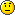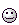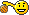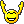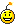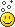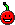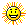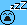Green Snake

Mensagens : 2185
Data de inscrição : 2012-04-07
Localização : I do not even know
Current Project : nopeJdpense

Mensagens : 100278
Data de inscrição : 2014-08-21Jdpense

Mensagens : 100278
Data de inscrição : 2014-08-21